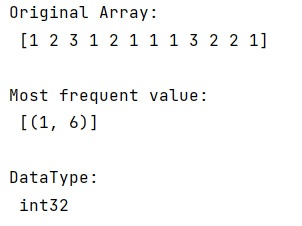# Find the most frequent value in a NumPy array

Learn, how to find the most frequent value in a NumPy array?
Submitted by Pranit Sharma, on December 24, 2022

NumPy is an abbreviated form of Numerical Python. It is used for different types of scientific operations in python. Numpy is a vast library in python which is used for almost every kind of scientific or mathematical operation. It is itself an array which is a collection of various methods and functions for processing the arrays.

## Finding the most frequent value in a NumPy array

Mathematically, the most frequent value in a number series is called its mode. Here, we are given a NumPy array and we need to find the most frequent element in this NumPy array.

For this purpose, we will first use the counter() method of the collections library and then we will apply the most_common() attribute of the counter() method.

Let us understand with the help of an example,

## Python code to find the most frequent value in a NumPy array

```# Import counter from collections
from collections import Counter

# Import numpy
import numpy as np

# Creating a numpy array
arr = np.array([1,2,3,1,2,1,1,1,3,2,2,1])

# Display original array
print("Original Array:\n",arr,"\n")

# count values
count = Counter(arr)

# Most frequent value
res = count.most_common(1)

# Display result
print("Most frequent value:\n",res,"\n")
```

Output: